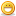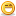# Thread: LED's and Resistors Guide

1. ##LED's and Resistors Guide

Led and Resistor Walkthru

__________________________________________________ __________________________________________________ _________________________

I wrote this little bit of information to help out. I by no means am an electrical engineer but I have tested this and am not responsible for any damage done to anything you take from this mod walkthru. I also realize there is many other calculators out there but didn't seem to find a walkthru here so posted my old one that I wrote up a long time ago so that people could figure it out on their own.

There is 2 power lines on the computer power supply on the standard molex style cables. That is the 5 volt line which is the red wire and the 12 volt wire which is the yellow.

First off I am going to show a simple equation. The equation to figure out what type of resistor is needed the equation is (power line - led volatge) / led amps. When you buy your led make sure you know the volatage required for it and the amps of it. Some leds might be 10mA while some might be 20mA or can vary.

Anyways now that we have the equation lets apply it.

So lets say we want to put 1 led on a 5 volt line and the led is 3.6 volts and has a milliamp of 20 then this is what that would look like. Also you have to convert the milliamps rating for the equation so devide the milliamps by 1000. For example a 20 Milliamp rating divided by 1000 = 0.02 Amps .

(5 volt[power] - 3.6 volt [led]) / 0.02 amps [ 20 mA]
= 70

So now we know you need a resistor that is 70 ohms

If this was a 12 volt line it would then be:

(12 volt[power] - 3.6 volt [led]) / 0.02 amps [ 20 mA]
= 420

So on the 12 volt rail you need a 420 ohm resistor.

Anyways that is the basics.

If you want to add more then 1 led then you times the volts of the LED by the number of LED's and use that number in your equation.

--------------------

This would be for putting them in series.

Example:

two 3.6 leds with a mA of 20 would be 7.2 volts and you would still be at 20mA so the equation looks like this.

(12volt[power] - 7.2 volt [led's]) / 0.02 amps [20 mA]
= 240

--------------------

This would be the equation for parallel.

Example:

three 3.6 leds with a mA of 20 on a 5 volt rail.

In parallel you multiply the mA by the number of Led's you want to use so since we want 3 leds it would be a mA of 60.

(5 volt[power] - 3.6[led]) / 0.06 amps [60 mA]
= 23

--------------------

The end results for the resistors won't be exact on with what you can buy so you need to find the closest one. As I mentioned I am not an eletrical engeneer so some of that might be a bit off but I have used these calculations many times in my mods and have not had an issue or anything blow up yet.Reply With Quote

2. ##Re: LED's and Resistors Guide

nice tutorial. now all you n00bs should figure out how to do the magic for the ubermodsReply With Quote

3. ##Re: LED's and Resistors Guide

nice, this is the perfect guide.. thanks for that whisp, im no rocket scientist and that guide put it down in plain english! taReply With Quote

4. ##Re: LED's and Resistors Guide

ok, one question... say i mess up and dont do it right, can i screw anything (other than the LED) by doing this?Reply With Quote

5. ##Re: LED's and Resistors Guide

No, unless you have something else in the circuit.
If you pour too much power into the LED, it will simply blow and close the circuit... And if by chance you manage to short out your power supply... modern PSU's (for about 5-10 years now) have short circuit protection... the other day, i short circuited my PSU while testing a tornado fan (damn those things have some torque)... the PSU was fine after i unplugged it and plugged it back inReply With Quote

6. ##Re: LED's and Resistors Guide

Might burn out the resistor on the setup of it but never seen any led go bad unless a lot of juice is put thru it.

Also depends on the power supply but most likely the power supply will just short and turn off. Thats not guranteed though.

I also think that just a burned out resistor and a led if it did would not cause the system to fail.

For example sometimes the leds on the front of your computers burn out and are still plugged in but the system still runs.

LED's are probabaly one of the easier electrical mods to start with.

As long as you have the proper resistor somewhere along the circuit of the item you are making since it dont matter if it comes at the end or at the beginning so sort of fail proof.

Just make sure you are attaching to the right voltage line with the appropriate resistors using the calculations and you should be good to go.Reply With Quote

7. ##Re: LED's and Resistors GuideOriginally Posted by Xato
a tornado fan (damn those things have some torque)...
they are f**king loud as well. I replaced mine with Red led quads. much quieter.Reply With Quote

8. ##Re: LED's and Resistors Guide

Lol, they sure can pass a **** load of air over your Heatsink though. Never use without a fan control though, or the noise will become unbearable.Reply With Quote

9. ##Re: LED's and Resistors Guide

ahhh good! that leaves a little room for 'trial and error'Reply With Quote

10. ##Re: LED's and Resistors Guide

hello, im new

when you say parallel, do you mean to place the resistor before all the LEDs or for each LED to get a resistor. I am betting on the first.. im unsure.. its been awhile..Reply With Quote